# S11E3 - Vapor Pressure of Solution, and Raoult's Law Calculations

We've already seen the vapor pressure of a pure liquid (solvent only) in SECTION 10 - Liquids, Solids, and Intermolecular Forces.

As a refresher, here's the vapor pressure of pure water: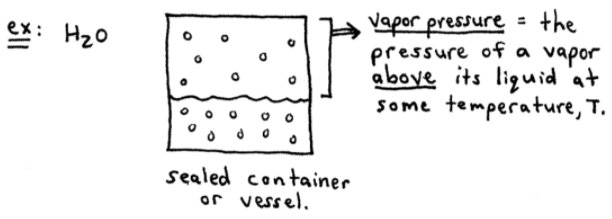vapor pressure  =  the pressure of a vapor above its liquid at some temperature, T.

----------

## Vapor Pressure of Solutions

Question:  What happens when you add a nonvolatile solute to a solvent, thus making a solution?

nonvolatile solute  =  a solute that has no tendecy to escape from solution into the gas phase (ex:  NaCl).Answer:  In the above sketch, the solution contains less molecules in the vapor (gas) phase relative to the pure solvent situation.

So, the presence of a nonvolatile solute lowers the vapor pressure (Pvap) of a solvent.

WHY ?

Because the dissolved nonvolatile solute decreases the number of solvent molecules per unit volume, and therefore lowers the escaping tendecy of the solvent molecules.

Also, occasionally solute molecules will "block" the surface by which solvent molecules must "break through" en route to the gas phase.

==========

## Raoult's Law

Raoult's Law  =  shows that the addition of a nonvolatile solute simply "dilutes" the solvent:----------

## Vapor Pressure Calculations

Let's use Raoult's Law in a few Vapor Pressure Calculations and sample problems...

ex:  Calculate the vapor pressure at 25°C for a solution made by dissolving 158.0g of sucrose (MM = 342.3 g/mol) in 643.5 cm3 water.  At 25°C, the vapor pressure of H2O is 23.76 torr and its density is 0.9971 g/cm3.
_________

➞  we need to find the V.P. of the solution, Pvap

### Psol'n  =  XH2O . P°H2O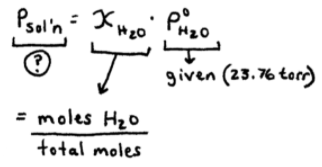So, we need the moles of H2O and the moles of sucrose, which together will give us the total moles: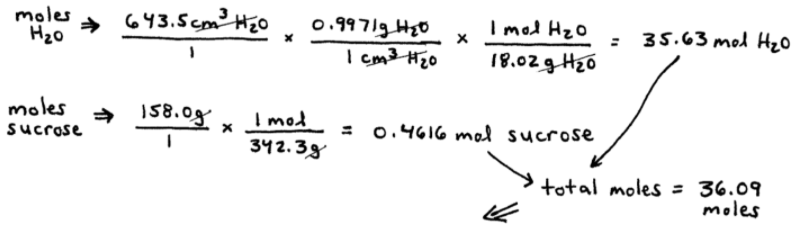Now we can calculate the mole fraction for water, XH2O, and finally the vapor pressure of solution, Pvap ...Notice that adding a solute (sucrose) lowered the vapor pressure by 0.30 torr.

Psol'n  -  PH2O  =  0.30 torr
23.76 torr  -  23.46 torr  =  0.30 torr

==========

ex:  Find the vapor pressure of a solution made by mixing 35.0 g of Na2SO4 (MM = 142 g/mol) with 175g of H2O at 25°C.  The vapor pressure of pure H2O is 23.76 torr at 25°C.
_________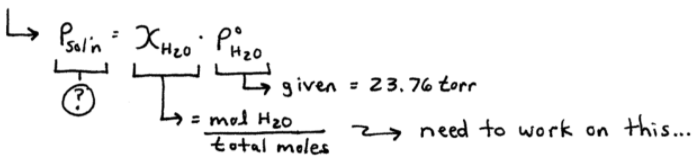So, we need the moles of H2O and the moles of ions that Na2SO4 dissociates into, which all together will give us the total moles:Now we can calculate the mole fraction for water, XH2O, and finally the vapor pressure of solution, Pvap ...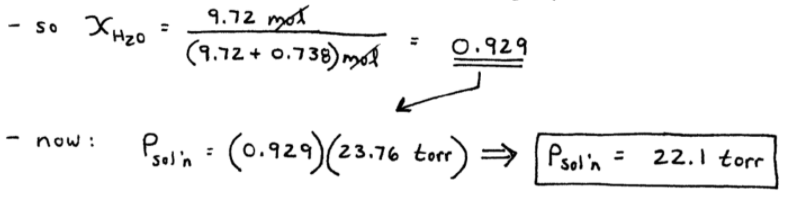Adding 35.0 g of sodium sulfate (Na2SO4) lowered the vapor pressure of pure water from 23.76 torr to 22.1 torr.

==========

Okay, next up in SECTION 11 - Solutions and Their Properties,

We'll discuss:

See you soon!  ✔️